Next: Parallel Algorithm Analysis Up: Scalable Data Parallel Algorithms Previous: Scalable Data Parallel Algorithms

# Introduction

Random Fields have been successfully used to sample and synthesize textured images (, , , , , , , , , , , , , , ). Texture analysis has applications in image segmentation and classification, biomedical image analysis, and automatic detection of surface defects. Of particular interest are the models that specify the statistical dependence of the gray level at a pixel on those of its neighborhood. There are several well-known algorithms describing the sampling process for generating synthetic textured images, and algorithms that yield an estimate of the parameters of the assumed random process given a textured image. Impressive results related to real-world imagery have appeared in the literature (, , , , , , , ). However, all these algorithms are quite computationally demanding because they typically require on the order of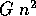arithmetic operations per iteration for an image of size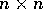with G gray levels. The implementations known to the authors are slow and operate on images of size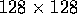or smaller. Recently, a parallel implementation has been developed on a DAP 510 computer . However, the DAP requires the structure of the algorithms to match its topology, and hence the corresponding algorithms are not as machine-independent as the algorithms described in this paper. In addition, we show that our algorithms are scalable in machine size and problem size.

In this paper, we develop scalable data parallel algorithms for implementing the most important texture sampling and synthesis algorithms. The data parallel model is an architecture-independent programming model that allows an arbitrary number of virtual processors to operate on large amounts of data in parallel. This model has been shown to be efficiently implementable on both SIMD (Single Instruction stream, Multiple Data stream) or MIMD (Multiple Instruction stream, Multiple Data stream) machines, shared-memory or distributed-memory architectures, and is currently supported by several programming languages including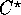, data-parallel C, Fortran 90, Fortran D, and CM Fortran. All our algorithms are scalable in terms of the number of processors and the size of the problem. All the algorithms described in this paper have been implemented and thoroughly tested on a Connection Machine CM-2 and a Connection Machine CM-5.

The Thinking Machines CM-2 is an SIMD machine with 64K bit-serial processing elements (maximal configuration). This machine has 32 1-bit processors grouped into a Sprint node to accelerate floating-point computations, and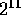Sprint nodes are configured as an 11-dimensional hypercube. See Figure 1 for the organization of a Sprint node.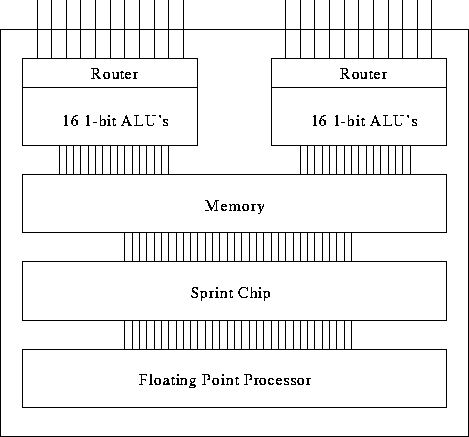Figure: The organization of a CM-2 Sprint node

The Thinking Machines CM-5 is a massively parallel computer with configurations containing 16 to 16,384 sparc processing nodes, each of which has four vector units, and the nodes are connected via a fat-tree communications network. The CM-5 is an MIMD machine which can run in Single Program Multiple Data (SPMD) mode to simulate SIMD operation. An in-depth look at the network architecture of this machine is described in . The nodes operate in parallel and are interconnected by a fat-tree data network. The fat-tree resembles a quad-tree, with each processing node (PN) as a leaf and data routers at all internal connections. In addition, the bandwidth of the fat-tree increases as you move up the levels of the tree towards the root. Leiserson () discusses the benefits of the fat-tree routing network, and Greenberg and Leiserson () bound the time for communications by randomized routing on the fat-tree. In this paper, we assume the Single Program Multiple Data (SPMD) model of the CM-5, using the data parallel language. In the SPMD model, each processing node executes a portion of the same program, but local memory and machine state can vary across the processors. The SPMD model efficiently simulates the data parallel SIMD model normally associated with massively parallel programming. References  and  provide an overview for the CM-5, and both \ and  contain detailed descriptions of the data parallel platform. Note that a CM-5 machine with vector units has four vector units per node, and the analysis given here will remain the same. See Figure 2 for the general organization of the CM-5 with vector units.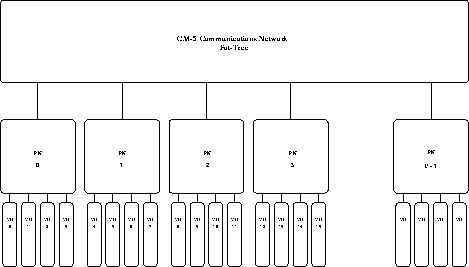Figure: The organization of the CM-5

This paper addresses a simple image processing problem of texture synthesis and compression using a Gibbs sampler as an example to show that these algorithms are indeed scalable and fast on parallel machines. Gibbs sampler and its variants are useful primitives for larger applications such as image compression (, ), image estimation (, , ), and texture segmentation (, , , , ).

Section 2 presents our model for data parallel algorithm analysis on both the CM-2 and the CM-5. In Section 3, we develop parallel algorithms for texture synthesis using Gibbs and Gaussian Markov Random Fields. Parameter estimation for Gaussian Markov Random Field textures, using least squares, as well as maximum likelihood techniques, are given in Section 4. Section 5 shows fast parallel algorithms for texture compression using the maximum likelihood estimate of parameters. Conclusions are given in Section 6.Next: Parallel Algorithm Analysis Up: Scalable Data Parallel Algorithms Previous: Scalable Data Parallel Algorithms

David A. Bader
dbader@umiacs.umd.edu